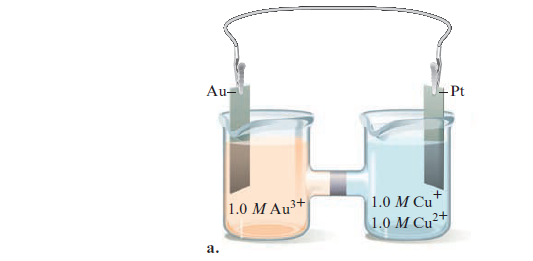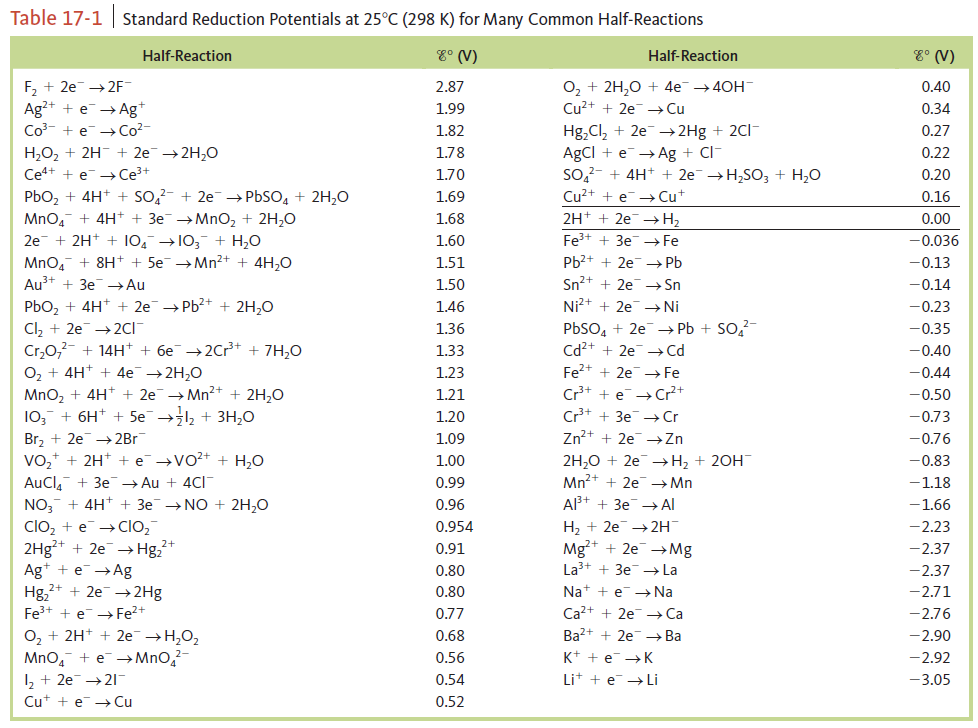# Problem: Consider the following galvanic cells:For each galvanic cell, give the balanced cell equation and determine E°. Standard reduction potentials are found in Table 17‑1.

###### FREE Expert Solution

Step 1

Au3+ + 3 e- Au E° = 1.50 V  E  reduction  cathode

Cu2+ + e Cu+ E° = 0.34   oxidation  anode

Step 2

Au3+ + 3 e- Au

[Cu+  Cu2+ + e-] x3

84% (44 ratings)###### Problem Details

Consider the following galvanic cells:For each galvanic cell, give the balanced cell equation and determine E°. Standard reduction potentials are found in Table 17‑1.Frequently Asked Questions

What scientific concept do you need to know in order to solve this problem?

Our tutors have indicated that to solve this problem you will need to apply the Cell Potential concept. If you need more Cell Potential practice, you can also practice Cell Potential practice problems.

What professor is this problem relevant for?

Based on our data, we think this problem is relevant for Professor Bernskoetter's class at MIZZOU.

What textbook is this problem found in?

Our data indicates that this problem or a close variation was asked in Chemistry: An Atoms First Approach - Zumdahl Atoms 1st 2nd Edition. You can also practice Chemistry: An Atoms First Approach - Zumdahl Atoms 1st 2nd Edition practice problems.